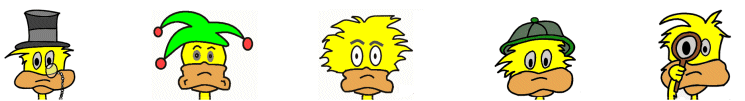# Kids Math

## Glossary and Terms: Operations

Example: 5 + 3 = 8, the addends are 5 and 3

Addition - The mathematical operation where two or more numbers are combined to make a sum. The "+" sign is used to indication addition.

Example: 4 + 2 + 7 = 13

Common factor - A factor that is shared by two or more numbers.

Example: The numbers 12 and 9 share the common factors 1 and 3. The number 4 is a factor of 12, but not 9 so it is not a common factor.

Dividend - When dividing, the dividend is the number that is getting divided or broken up.

Example: 12 ÷ 4 = 3, in this operation 12 is the dividend.

Divisible - A number is divisible if it can be divided without leaving a remainder.

Example: 12 ÷ 4 = 3. In this case, 12 is divisible by 4.

Division - A mathematical operation where a number is split into equal groups.

Example: 12 ÷ 4 = 3. In this example 12 is split into 4 equal parts of 3.

Divisor - In division, the divisor is the number by which the dividend is divided.

Example: 12 ÷ 4 = 3. In this example the 4 is the divisor.

Factors - The numbers that are multiplied together to get an answer in a multiplication operation.

Example: 4 x 6 = 24, the numbers 4 and 6 are factors.

Greatest common factor - The largest number that is a common factor of two numbers.

Example: The numbers 12 and 9 share the common factors 1 and 3. The number 3 is the greatest common factor.

Minuend - In a subtraction operation, the minuend is the number from which another number is subtracted.

Example: 40 - 10 = 30, in this operation 40 is the minuend.

Multiple - A multiple is the product of any number and another whole number.

Example: 3, 6, 9, 12, and 15 are all multiples of 3

Multiplication - The mathematical operation of scaling one number by another. It can be thought of as adding the same number together a given number of times. The symbol "x" is often used to indicate a multiplication operation.

Example: 5 x 3 = 15, this can also be written as 5 + 5 + 5 = 15.

Product - The product is the answer to a multiplication operation.

Example: 5 x 3 = 15, in this example 15 is the product.

Quotient - The quotient is the answer to a division operation. The quotient does not include the remainder, if there is one.

Example: 12 ÷ 4 = 3. In this operation, 3 is the quotient.

Remainder - The remainder is the amount left over after a division operation.

Example: 22 ÷ 4 = 5 r 2. In this example the remainder is 2.

Square - An operation where a number is multiplied by itself. It is written with a small 2 to the right of the number like X2.

Example: 52 = 5 x 5 = 25

Square root - A number that produces a given number when multiplied by itself. The symbol for square root is √.

Example: √ 25 = 5, 5 is the square root of 25 because 5 x 5 = 25.

Subtraction - A mathematical operation that solves for the difference between two numbers. The symbol for the operation is "-", which is also called the "minus" sign.

Example: 8 - 2 = 6

Subtrahend - This is the number that is subtracted in a subtraction operation.

Example: 8 - 2 = 6, in this example 2 is the subtrahend.

Example: 8 + 7 = 15, in this example 15 is the sum.

More Math Glossaries and Terms

Algebra glossary
Angles glossary
Figures and Shapes glossary
Fractions glossary
Graphs and lines glossary
Measurements glossary
Mathematical operations glossary
Probability and statistics glossary
Types of numbers glossary
Units of measurements glossary

Back to Kids Math

Back to Kids Study